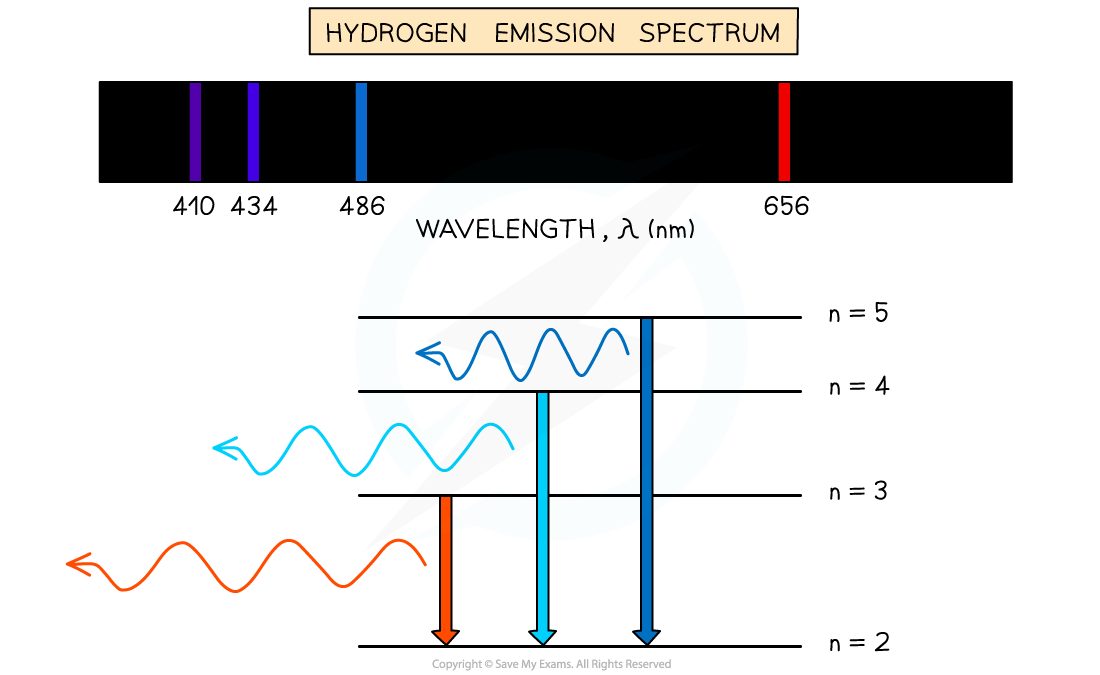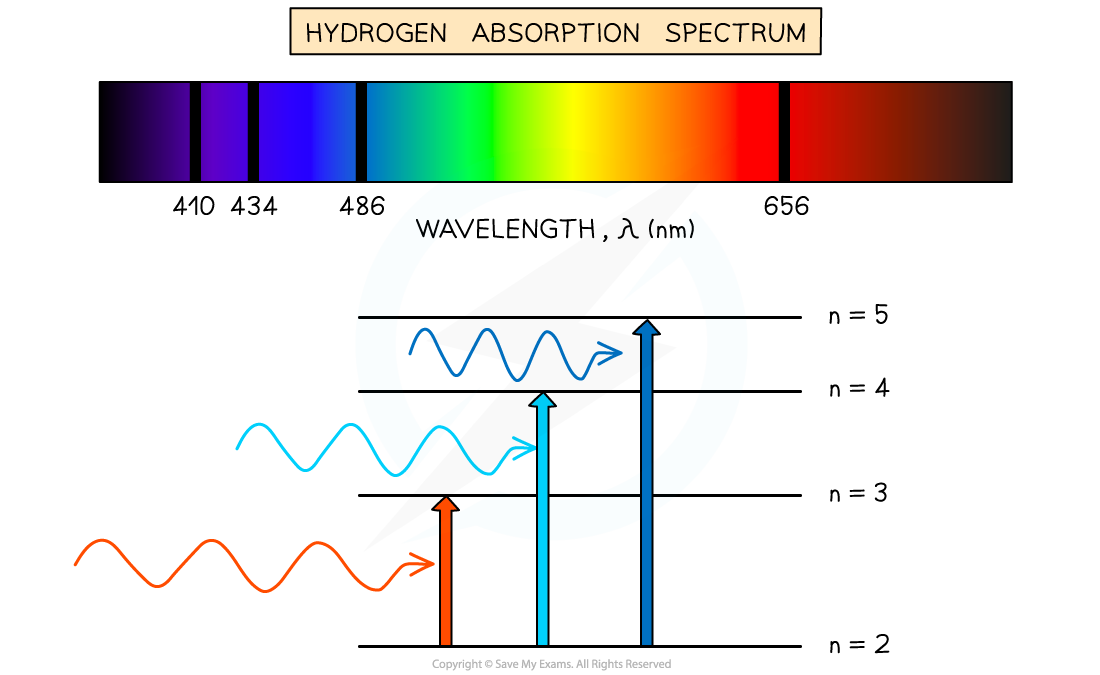# CIE A Level Physics复习笔记22.3.2 Line Spectra

### Line Spectra

• Line spectra is a phenomenon which occurs when excited atoms emit light of certain wavelengths which correspond to different colours
• The emitted light can be observed as a series of coloured lines with dark spaces in between
• These series of coloured lines are called line or atomic spectra
• Each element produces a unique set of spectral lines
• No two elements emit the same set of spectral lines, therefore, elements can be identified by their line spectrum
• There are two types of line spectra: emission spectra and absorption spectra

#### Emission Spectra

• When an electron transitions from a higher energy level to a lower energy level, this results in the emission of a photon
• Each transition corresponds to a different wavelength of light and this corresponds to a line in the spectrum
• The resulting emission spectrum contains a set of discrete wavelengths, represented by coloured lines on a black background
• Each emitted photon has a wavelength which is associated with a discrete change in energy, according to the equation:• Where:
• Δ= change in energy level (J)
• h = Planck’s constant (J s)
• f = frequency of photon (Hz)
• c = the speed of light (m s-1)
• λ = wavelength of the photon (m)
• Therefore, this is evidence to show that electrons in atoms can only transition between discrete energy levelsThe emission spectrum of hydrogen

#### Absorption Spectra

• An atom can be raised to an excited state by the absorption of a photon
• When white light passes through a cool, low pressure gas it is found that light of certain wavelengths are missing
• This type of spectrum is called an absorption spectrum
• An absorption spectrum consists of a continuous spectrum containing all the colours with dark lines at certain wavelengths
• These dark lines correspond exactly to the differences in energy levels in an atom
• When these electrons return to lower levels, the photons are emitted in all directions, rather than in the original direction of the white light
• Therefore, some wavelengths appear to be missing
• The wavelengths missing from an absorption spectrum are the same as their corresponding emission spectra of the same elementThe absorption spectrum of hydrogen